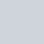# Variance and Standard Deviation in Statistics

•
•
•
•
•
•
•
•
•

Variance is one of the best measure of dispersion which measure the difference of all observation from the center value of the observations.

### Population variance and standard deviation

The average of the square of the deviations taken from mean is called variance. The population variance is generally denoted by σ2and its estimate (sample variance) by s2. For N population values X1,X2,…,XN having the population mean μ, the population variance is defined as,

Where,  μ is the mean of all the observations in the population and N is the total number of observations in the population. Because the operation of squaring, the variance is expressed in square units and not of the original units.

So, we can define the population standard deviation as,

Thus, the standard deviation is the positive square root of the mean square deviations of the observations from their arithmetic mean. More simply, standard deviation is the positive square root of σ2.

### Sample variance

In maximum statistical applications, we deal with a sample rather than a population. Thus, while a set of population observations yields a σ2 and a set of sample observations will yield a s2. If x1,x2,…,xn is a set of sample observations of size n, then the s2 is define as,

### Properties

Effect of changes in origin: Variance and statndard deviation have certain appealing properties. Let each of the numbers x1,x2,…,xn increases or decreases by a constant c. Let y be the transformed variable defined as,

where, c is a constant.
Finally we get that any linear change in the variable x does not have any effect on its σ2. So, σ2 is independent of change of origin.

Effect of changes in the scale: When each observation of the variable is multiplied or divided by a certain constant c then there occur changes in the σ2.

So, we can say that, Changes in scale affects and it depends on scale.

### Uses of Variance and standard deviation

A thorough understanding of the uses of standard deviation is difficult for us as this stage, unless we acquire some knowledge on some theoritical distributions in statistics. The variance and standard deviation of a population is a measure of the dispersion in the population while the variance and standard deviation of sample observations is a measure of the dispersion in the distribution constructed from the sample. It can be the best understood with reference to a normal distribution because normal distribution is completely defined by mean and standard deviation.

### 5 thoughts on “Variance and Standard Deviation in Statistics”

1.I Fashion Styles

Good day very cool blog!! Guy .. Beautiful .. Superb .. I will bookmark your site and take the feeds additionally. I am happy to seek out so many helpful info right here within the publish.

2.https://www.wheretoskiandsnowboard.com/?URL=https://dailiest.com

It’s very easy to find out any topic on net as compared to books,
as I found this paragraph at this web site.

3.Clarissa Nottingham

Excellent weblog here! Additionally your web site rather a lot up fast!
What web host are you the use of? Can I am getting your affiliate hyperlink in your
host? I wish my web site loaded up as quickly as yours lol

1.Statistical Aid

My hosting:
https://manage.xeonbd.com/aff.php?aff=689

4.Eneida Broderson

whoah this weblog is magnificent i love studying your posts.
Keep up the great work! You realize, lots of persons are searching around for this information, you could help them
greatly.